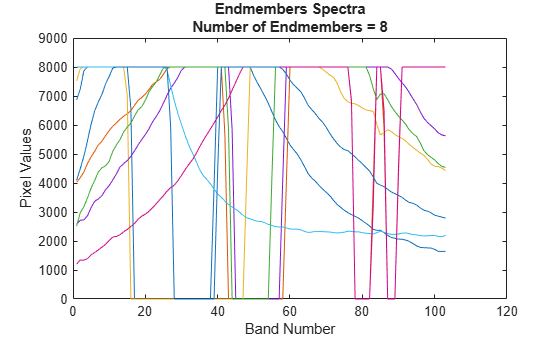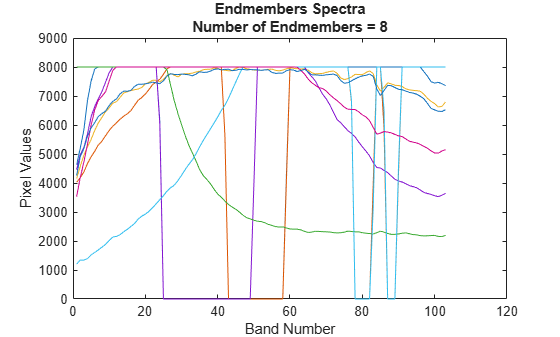# ppi

Extract endmember signatures using pixel purity index

Since R2020a

## Syntax

``endmembers = ppi(inputData,numEndmembers)``
``endmembers = ppi(inputData,numEndmembers,Name,Value)``

## Description

example

````endmembers = ppi(inputData,numEndmembers)` extracts endmember signatures from hyperspectral data `cube` by using the pixel purity index (PPI) algorithm. `numEndmembers` is the number of endmember signatures to be extracted using PPI algorithm.The function projects the hyperspectral data onto a set of randomly generated unit vectors. The pixels with extreme values in the direction of an unit vector are considered pure pixels and they constitute the endmembers. The value of an endmember across all the spectral bands in the input data comprises the endmember signature. For more information, see Algorithms.```

example

````endmembers = ppi(inputData,numEndmembers,Name,Value)` specifies options using one or more name-value pair arguments in addition to the input arguments in the previous syntax. Use this syntax to set the options for number of randomly generated unit vectors to be used for projection.extracting endmember signatures from a reduced hyperspectral data. NoteThis function requires the Image Processing Toolbox™ Hyperspectral Imaging Library. You can install the Image Processing Toolbox Hyperspectral Imaging Library from Add-On Explorer. For more information about installing add-ons, see Get and Manage Add-Ons.The Image Processing Toolbox Hyperspectral Imaging Library requires desktop MATLAB®, as MATLAB Online™ or MATLAB Mobile™ do not support the library. ```

## Examples

collapse all

Read a hyperspectral data into the workspace.

`hcube = hypercube('paviaU.hdr');`

Find the number of spectrally distinct endmembers present in the hyperspectral data cube by using `countEndmembersHFC` function.

`numEndmembers = countEndmembersHFC(hcube,'PFA',10^-7);`

Compute the endmembers using the pixel purity index (PPI) method. By default, the `ppi` function uses maximum noise fraction (MNF) transform for preprocessing. The default number of skewers used for projection is $1{0}^{4}$.

`endmembers = ppi(hcube.DataCube,numEndmembers);`

Plot the endmembers of the hyperspectral data.

```figure plot(endmembers) xlabel('Band Number') ylabel('Pixel Values') title({'Endmembers Spectra',['Number of Endmembers = ' num2str(numEndmembers)]}); ylim([0 9000])```Read a hyperspectral data into the workspace.

`hcube = hypercube('paviaU.hdr');`

Find the number of spectrally distinct endmembers present in the hyperspectral data cube by using `countEndmembersHFC` function.

`numEndmembers = countEndmembersHFC(hcube,'PFA',10^-7);`

Compute the endmembers using the pixel purity index (PPI) method. Specify the number of unit vectors to be used for projection as 100. Also, select principal component analysis (PCA) method for dimensionality reduction.

`endmembers = ppi(hcube.DataCube,numEndmembers,'NumVectors',100,'ReductionMethod','PCA');`

Plot the endmembers of the hyperspectral data.

```figure plot(endmembers) xlabel('Band Number') ylabel('Pixel Values') ylim([0 9000]) title({'Endmembers Spectra',['Number of Endmembers = ' num2str(numEndmembers)]});```## Input Arguments

collapse all

Input hyperspectral data, specified as an 3-D numeric array or a `hypercube` object. If the input is a `hypercube` object, then the function reads the hyperspectral data cube from its `DataCube` property.

The hyperspectral data is an numeric array of size M-by-N-by-C. M andN are the number of rows and columns in the hyperspectral data respectively. C is the number of spectral bands in the hyperspectral data.

Data Types: `single` | `double` | `int8` | `int16` | `int32` | `int64` | `uint8` | `uint16` | `uint32` | `uint64`

Number of endmembers to be extracted, specified as a positive scalar integer. The value must be in the range [`1` C]. C is the number of spectral bands in the input hyperspectral data.

Data Types: `single` | `double` | `int8` | `int16` | `int32` | `int64` | `uint8` | `uint16` | `uint32` | `uint64`

### Name-Value Arguments

Specify optional pairs of arguments as `Name1=Value1,...,NameN=ValueN`, where `Name` is the argument name and `Value` is the corresponding value. Name-value arguments must appear after other arguments, but the order of the pairs does not matter.

Before R2021a, use commas to separate each name and value, and enclose `Name` in quotes.

Example: `ppi(inputData,7,'NumVectors',100,'Method','None')`

Number of random unit vectors, specified as the comma-separated pair of '`NumVectors`' and a positive scalar integer. The accuracy of the extracted endmembers increases with the number of vectors used for projection. However, increasing the number of vectors also increases the computational complexity.

Data Types: `single` | `double` | `int8` | `int16` | `int32` | `int64` | `uint8` | `uint16` | `uint32` | `uint64`

Dimensionality reduction method, specified as the comma-separated pair of '`ReductionMethod`' and `'MNF'`, `'PCA'`, or `'None'`.

If you specify the value as `'MNF'` or `'PCA'`, the function first reduces the spectral dimension of the input data by using the specified method. Then, it computes the endmember signatures from the reduced data.

• `'MNF'` — perform dimensionality reduction using the maximum noise fraction (MNF) method.

• `'PCA'` — perform dimensionality reduction using the principal component analysis (PCA) method.

If you specify the value as `'None'`, the function does not perform dimensionality reduction. The endmember signatures are extracted directly from the input data.

Data Types: `char` | `string`

## Output Arguments

collapse all

Endmember signatures, returned as a matrix of size C-by-P and datatype same as the input hyperspectral data.

Data Types: `single` | `double` | `int8` | `int16` | `int32` | `int64` | `uint8` | `uint16` | `uint32` | `uint64`

## Algorithms

Pixel purity index (PPI) method computes the orthogonal projections of hyperspectral data values on a set of randomly generated unit vectors known as the skewers. Then, the method computes the PPI count for each data value. PPI count is the number of times a data value results as an extrema point when projected on to these skewers. Those data values with more than expected number of PPI count comprise the endmembers of the hyperspectral data. PPI is a non-iterative method and the steps involved are summarised as follows:

1. Compute principal component bands and reduce the dimensionality of the input data by using MNF or PCA. The number of principal component bands to be extracted is set equal to the number of endmembers to be extracted.

2. Generate k number of skewers of length same as the input data.

3. Let r be the sample vector that denote a pixel spectra. Then, orthogonally project the sample vector onto each skewers and find the extrema.

4. Store the location of each extreme value and count their occurrences. The number of occurrences is known as the PPI count.

5. Find the PPI count for each pixel spectra in the input data cube.

6. Arrange the pixel spectra in descending order of their PPI counts and identify the first n number of pixel spectra in the ordered set as endmembers. The number of endmembers to be selected is specified by the input argument `numEndmembers`.

 J.W Boardman, F.A. Kruse and R.O. Green, "Mapping target signatures via partial unmixing of AVIRIS data.", Technical Report, California, USA, 1995.